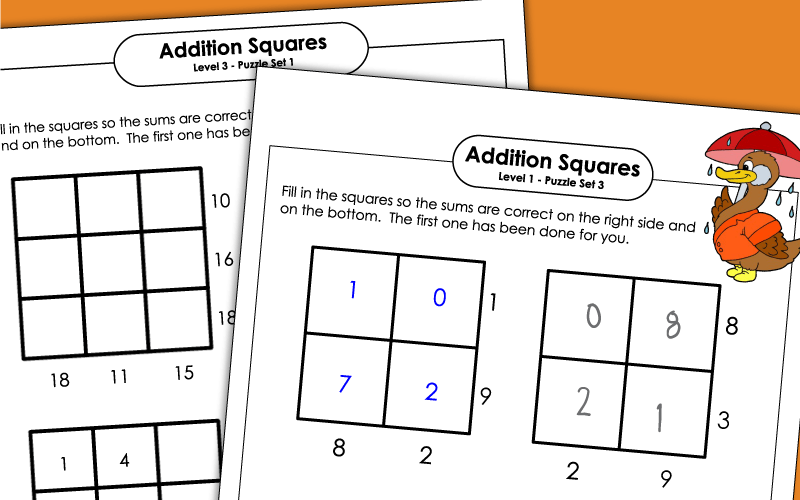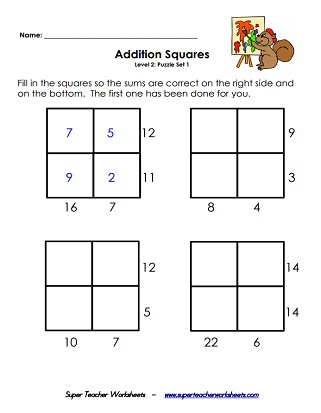# Addition Squares - Logic Puzzles

Printable addition square logic puzzles.  To solve, students make place numbers in the squares to make the given sums.## Level 1 (Easy)

Students complete the addition squares by putting numbers in boxes to make the given sums.  Easiest level.  2x2 grid.
More level 1 addition squares; Includes 3 puzzles for students to solve; Sums up to 18
Even more level level 1 addition puzzles to build students' logic skills.

## Level 2 (Intermediate)

More difficult addition squares.  Solve by placing numbers in boxes to find the given sums.  2x2 grid.
More addition square brain teasers for students to solve; Sums up to 30;  Medium level.

## Level 3 (Challenging)

Most difficult math addition squares on a 3x3 grid.  Challenging level.
Yet another set of addition puzzles on a 3 by 3 grid for students to solve.
Brain Teaser Worksheets

Baffling brain teaser worksheets

Algebra Worksheets

Learn about expressions, equations, and inequalities.

In and Out Boxes

Printable worksheets with input, output boxes

## Worksheet ImagesMy Account
Site Information